# High School Math : Radicals

## Example Questions

← Previous 1 3 4 5

### Example Question #1 : Understanding Radicals

Simplify the expression. Find the positive solution only.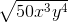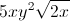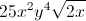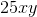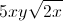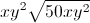Explanation:

When working in square roots, each component can be treated separately.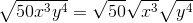Now, we can simplify each term.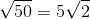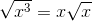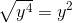Combine the simplified terms to find the answer. Anything outside of the square root is combined, while anything under the root is combined under the root.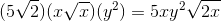### Example Question #2 : Radicals

Convert the radical to exponential notation.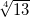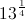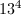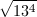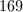Explanation:

Remember that any term outside the radical will be in the denominator of the exponent.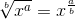Sincedoes not have any roots, we are simply raising it to the one-fourth power.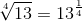### Example Question #1 : Expressing Radicals As Exponents

What is the value of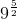?

2.41

27

243

9

3

243

Explanation:

An exponent written as a fraction can be rewritten using roots.can be reqritten as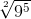.  The bottom number on the fraction becomes the root, and the top becomes the exponent you raise the number to.is the same as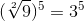. This will give us the answer of 243.

### Example Question #4 : Radicals

Express the following radical in rational (exponential) form: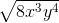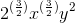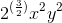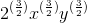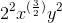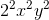Explanation:

To convert the radical to exponent form, begin by converting the integer: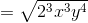Now, divide each exponent byto clear the square root: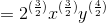Finally, simplify the exponents: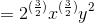### Example Question #5 : Radicals

Express the following radical in rational (exponential) form: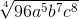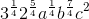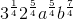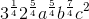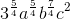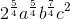Explanation:

To convert the radical to exponent form, begin by converting the integer: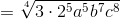Now, divide each exponent byto cancel the radical: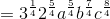Finally, simplify the exponents: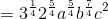### Example Question #6 : Radicals

Which fraction is equivalent to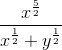?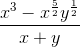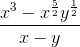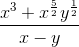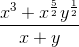Explanation:

Multiply the numerator and denominator by the compliment of the denominator: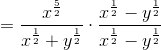Simplify the expression: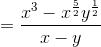### Example Question #7 : Radicals

Simplify the following radical. Express in rational (exponential) form.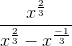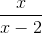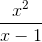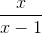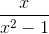Explanation:

Multiply the numerator and denominator by the compliment of the denominator: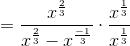Simplify the expression: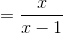### Example Question #8 : Radicals

Choose the fraction equivalent to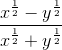.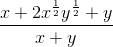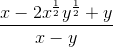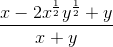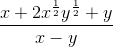Explanation:

Multiply the numerator and denominator by the compliment of the denominator: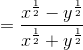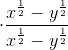Simplify the expression: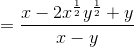### Example Question #9 : Radicals

Simplify the following radical. Express in rational (exponential) form.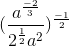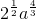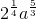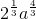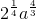Explanation:

Multiply the numerator and denominator to the exponent: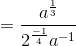Simplify the expression by combining like terms: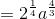### Example Question #10 : Radicals

Express the following radical in rational (exponential) form: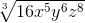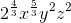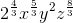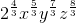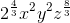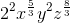Explanation:

To convert the radical to exponent form, begin by converting the integer: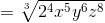Now, divide each exponent byto remove the radical: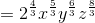Finally, simplify the exponents: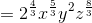← Previous 1 3 4 5